# Class 12 RD Sharma Solutions – Chapter 30 Linear Programming – Exercise 30.4 | Set 3

• Last Updated : 02 Jun, 2021

### Question 35. A manufacturer makes two products, A and B. Product A sells at Rs 200 every and takes 1/2 hour to make. Product B sells at Rs 300 each and takes 1 hour to make. There is a permanent order for 14 units of product A and 16 units of product B. A working week consists of 40 hours of production and the weekly turnover must not be less than Rs 10000. If the profit on each of product A is Rs 20 and a product B is Rs 30, then how many of each should be produced so that the profit is maximum? Also, find the maximum profit.

Solution:

Assume x units of product A and y units of product B were manufactured.

Attention reader! All those who say programming isn't for kids, just haven't met the right mentors yet. Join the  Demo Class for First Step to Coding Coursespecifically designed for students of class 8 to 12.

The students will get to learn more about the world of programming in these free classes which will definitely help them in making a wise career choice in the future.

Number of units cannot be negative.

Therefore,

x, y ≥ 0 (always)

As per the question, the given information can be shown as

Also, the availability of time is 40 hours and the revenue should be at least Rs 10000.

So, further, it is given that there is a permanent order for 14 units of product A and 16 units of product B.

Therefore, the constraints are

200x + 300y ≥ 100000,

5x + y ≤ 40

x ≥ 14

y ≥ 16

If the profit on each of product A is Rs 20 and on product B is Rs 30.

Therefore, profit gained on x units of product A and y units of product B is Rs 20x and Rs 30y respectively.

Total profit = Z = 20x + 30y which is to be maximised

Therefore, the formula of the given linear programming problem is

Max Z = 20x + 30y

Subject to constraints

2x + 3y ≥ 100

x + 2y ≤ 80

x ≥ 14

y ≥ 16

x, y ≥ 0

First we will convert in-equations into equations so follows:

2x + 3y = 100,

x + 2y = 80,

x = 14,

y = 16,

x = 0 and

y = 0.

Area shows by 2x + 3y ≥ 100

The line 2x + 3y = 100 connects the coordinate axes at A(50, 0) and B(0, 100/3) respectively.

By connecting these points we will get the line 2x + 3y = 100 .

So, (0, 0) does not assure the 2x + 3y = 100.

So, the area which does not have the origin shows the solution set of the in-equation 2x + 3y ≥ 100.

Area shows by x + 2y ≤ 80:

The line x + 2y = 80 connects the coordinate axes at C(80, 0) and D(0, 40) respectively.

By connecting these points we will get the line x + 2y = 80.

So, (0, 0) assure the in-equation x + 2y ≤ 80.

So, the area which haves the origin shows the solution set of the in-equation x + 2y ≤ 80.

Area shown by x ≥ 14

x = 14 is the line passes through 14, 0 and is parallel to the Y axis.

The area to the right of the line x = 14 will assure the in-equation.

Area shown by y ≥ 16

y = 16 is the line passes through 0, 16 and is parallel to the X axis.

The area above the line y = 16 will assure the in-equation.

Area shows by x ≥ 0 and y ≥ 0:

Since, every point in the first quadrant assure these in-equations.

So, the first quadrant is the area shows by the in-equations x ≥ 0, and y ≥ 0.

The suitable area determined by the system of constraints

2x + 3y ≥ 100,

x + 2y ≤ 80,

x ≥ 14,

y ≥ 16,

x ≥ 0 and

y ≥ 0 are so follows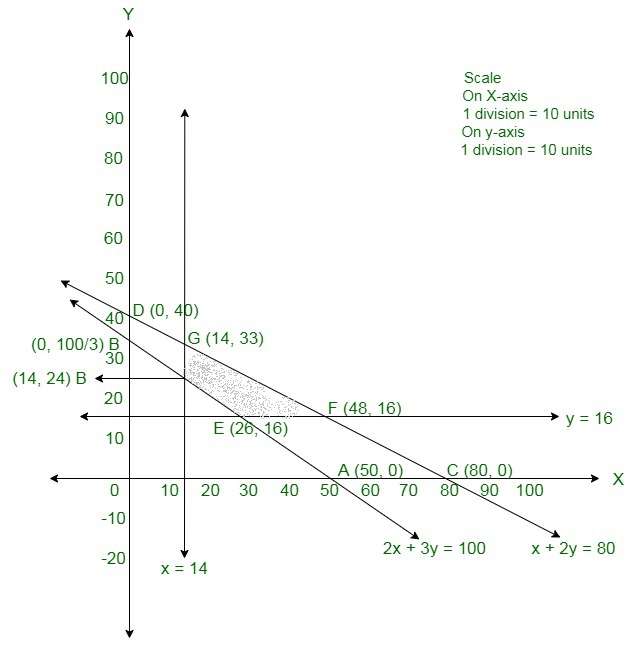The corner points of the suitable area are E(26, 16), F(48, 16), G(14, 33) and H(14, 24)

The values of Z at these corner points are so follow

The maximum value of Z is Rs 1440 which is obtained at F(48, 16).

Therefore,

The maximum profit is Rs 1440 will get when 48 units of product A and 16 units of product B were manufactured.

### Question 36. If a young man drives his vehicle at 25 km/hr, he has to spend 2 per km on petrol. If he drives it at a faster speed of 40 km/hr, the petrol cost increases to 5 per km. He has 100 to spend on petrol and travel within one hour. Express this to an LPP and solve the same.

Solution:

Assume that the man travels x km when the speed is 25 km/hour and y km when the speed is 40 km/hour.

Therefore, the total distance traveled is (x + y) km.

Now, it is given that the man has Rs 100 to spend on petrol.

Total cost of petrol = 2x + 5y ≤ 100

Now, time taken to travel x km = x/25 h

Time taken to travel y km = y/40 h

Now, it is given that the maximum time is 1 hour.

So,

x/25 + y/40 ≤ 1

⇒ 8x + 5y ≤ 200

Therefore, the formula of the given linear programming problem is

Maximise Z = x + y

Subject to the constraints

2x + 5y ≤ 100

8x + 5y ≤ 200

x ≥ 0, y ≥ 0

The suitable area determined by the given constraints can be diagrammatically shown as,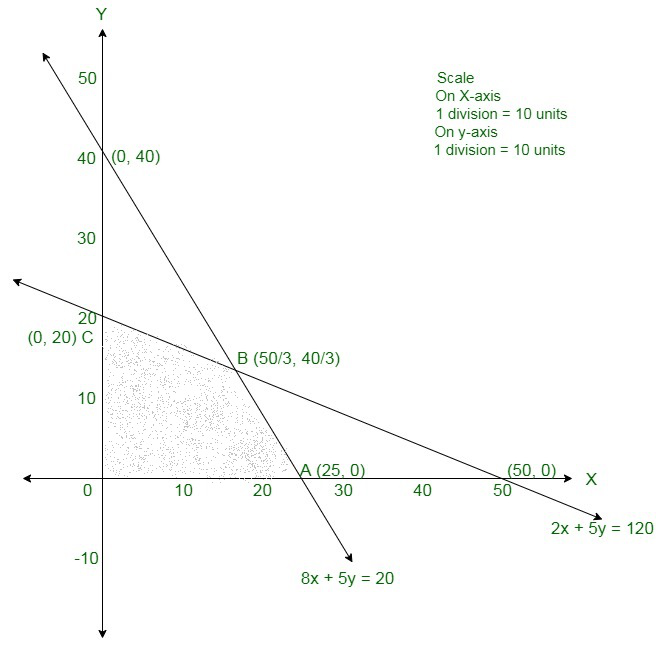The coordinates of the corner points of the suitable area are O(0, 0), A(25, 0), B(50/3, 40/3) and C(0, 20).

The value of the objective function at these points are given in the following table

So, the maximum value of Z is 30 at x = 50/3 , y = 40/3.

Therefore, the maximum distance that the man can travel in one hour is 30 km.

Therefore, The distance travelled by the man at the speed of 25 km/hour is 50/3 km, and the distance travelled by him at the speed of 40 km/hour is 40/3 km.

### Assuming that the transportation cost per km is Rs 1.00 per litre, how should the delivery be scheduled in order that the transportation cost is minimum?

Solution:

Assume x and y litres of oil be supplied from A to the petrol pumps D and E.

Then, (7000 − x − y) L will be supplied from A to petrol pump F.

The requirement at petrol pump D is 4500 L.

Since, x L are transported from depot A, the remaining (4500 −x) L will be transported from petrol pump B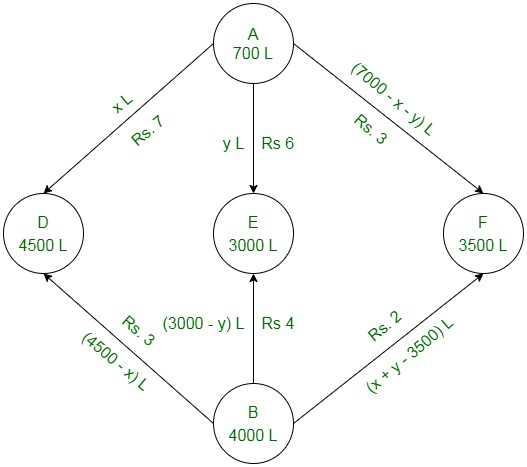Similarly, (3000 − y) L and [3500 − (7000 − x − y)] L i.e. (x + y − 3500) L will be transported from depot B to petrol pump E and F. respectively.

The given problem can be shown diagrammatically, so follows

Since the quantity of oil is non-negative, therefore,

x ≥ 0, y ≥ 0, and (7000 – x – y) ≥ 0

x ≥ 0,

y ≥ 0 and

x + y ≤ 7000

4500 – x ≥ 0, 3000 – y ≥ 0, and x + y – 3500 ≥ 0

x ≤ 4500,

y ≤ 3000, and

x + y ≥ 3500

Cost of transporting 10 L of petrol = Re 1

Cost of transporting 1 L of petrol = Rs 1/10

Thus, total transportation cost is given by,

z = 7/10 × x + 6/10y + 3/10(7000 – x – y) + 3/10(4500 – x) + 4/10(3000 – y) + 2/10(x + y – 3500)

= 0.3x + 0.1y + 3950

Therefore, the formula of the given linear programming problem is

Minimize Z = 0.3x + 0.1y + 3950

Subject to the constraints,

x + y ≤ 7000

x ≤ 4500

y ≤ 3000

x + y ≥ 3500

x, y ≥ 0

First we will convert in-equations into equations so follows:

x + y = 7000,

x = 4500,

y = 3000,

x + y = 3500,

x = 0 and

y = 0

Area shows by x + y ≤ 7000:

The line x + y = 7000 connects the coordinate axes at A(7000, 0) and B(0, 7000) respectively.

By connecting these points we will get the line x + y = 7000 .

So, (0, 0) assure the x + y = 7000 .

So, the area which haves the origin shows the solution set of the in-equation x + y ≤ 7000.

Area shown by x ≤ 4500:

The line x = 4500 is the line posses through C(4500, 0) and is parallel to Y axis.

The area to the left of the line x = 4500 will assure the in-equation x ≤ 4500.

Area shows by y ≤ 3000:

The line y = 3000 is the line passes through D(0, 3000) and is parallel to X axis.

The area below the line y = 3000 will assure the in-equation y ≤ 3000.

Area shows by x + y ≥ 3500:

The line x + y = 7000 connects the coordinate axes at E13500, 0 and F1(0, 3500) respectively.

By connecting these points we will get the line x + y = 3500 .

So, (0, 0) assure the x + y = 3500.

So, the area which have the origin shows the solution set of the in-equation

x + y ≥ 3500.

Area shown by x ≥ 0 and y ≥ 0:

Since, every point in the first quadrant assure these in-equations.

So, the first quadrant is the area shows by the in-equations x ≥ 0, and y ≥ 0.

The suitable area determined by the system of constraints

x + y ≤ 7000,

x ≤ 4500,

y ≤ 3000,

x + y ≥ 3500,

x ≥ 0 and

y ≥ 0 are so follows.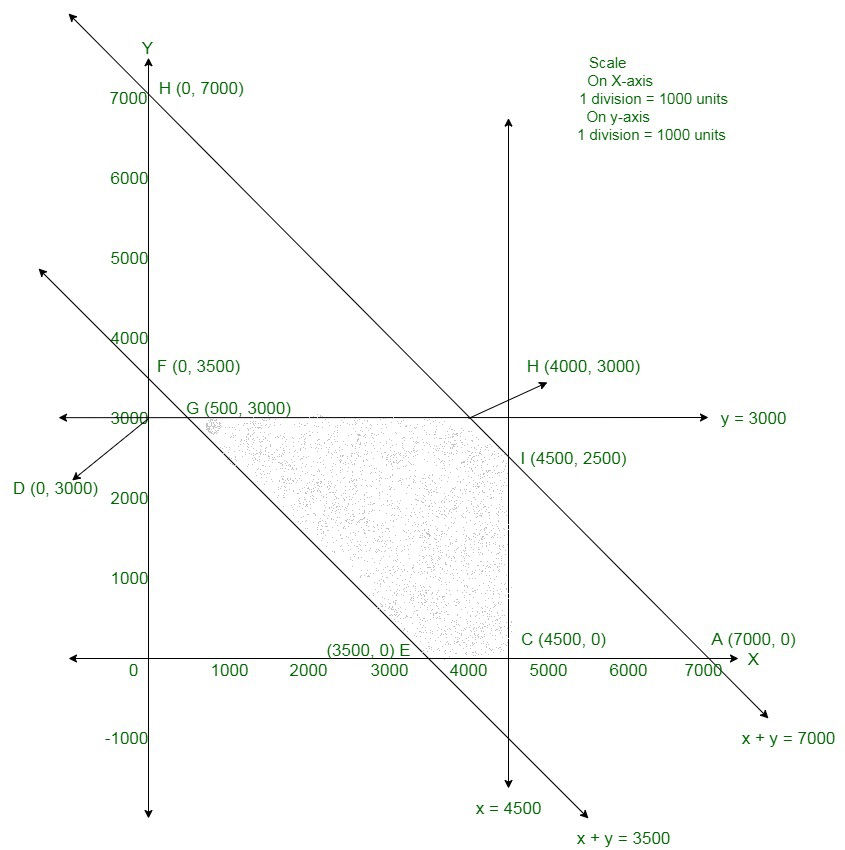The corner points of the suitable area are E(3500, 0), C(4500, 0), I(4500, 2500), H(4000, 3000), and G(500, 3000).

The values of Z at these corner points are so follows.

The minimum value of Z is 4400 at G (500, 3000).

Therefore, the oil supplied from depot A is 500 L, 3000 L, and 3500 L and from depot B is 4000 L, 0 L, and 0 L to petrol pumps D, E, and F respectively.

So, the minimum transportation cost is Rs 4400.

### Question 38. A small firm manufactures gold rings and chains. The total number of rings and chains manufactured per day is at most 24. It takes 1 hour to make a ring and 30 minutes to make a chain. The maximum number of hours available per day is 16. If the profit on a ring is Rs 300 and that on a chain is Rs 190, find the number of rings and chains that should be manufactured per day, so so to earn the maximum profit. Make it so an LPP and solve it graphically.

Solution:

Assume the firm manufacture x gold rings and y chains per day.

Number of gold rings and chains cannot be negative.

Therefore,

x, y ≥0 (always)

It is given that the total number of gold rings and chains manufactured per day is at most 24.

Therefore,

x + y ≤ 24

The gold ring takes 1 hour to make and chain takes 30 min, that is, 0.5 hour to make.

The maximum number of hours available per day is 16 hours.

Therefore,

x + 0.5y ≤ 16

The profit on a ring is Rs 300 and on a chain is Rs 190.

Therefore, profit made from x gold rings and y chains is Rs 300x and Rs 190y respectively.

Therefore,

Total profit, Z = 300x + 190y

Therefore, the formula of the given linear programming problem is

Maximise Z = 300x + 190y

Subject to the constraints

x + y ≤ 24

x + 0.5y ≤ 16

x, y ≥0

First we will convert in-equations into equations so follows:

x + y = 24,

x + 0.5y = 16,

x = 0 and y = 0

Area shown by x + y ≤ 24:

The line x + y = 24 connects the coordinate axes at A(24, 0) and B(0, 24) respectively.

By connecting these points we will get the line x + y = 24.

So, (0, 0) assure the x + y = 24. So, the area which have the origin shows the solution set of the in-equation

x + y ≤ 24.

Area shown by x + 0.5y ≤ 16:

The line x + 0.5y = 16 connects the coordinate axes at C(16, 0) and D(0, 32) respectively.

By connecting these points we will get the line x + 0.5y = 16.

So, (0, 0) assure the in-equation x + 0.5y ≤ 16.

So,the area which haves the origin shows the solution set of the in-equation x + 0.5y ≤ 16.

Area shows by x ≥ 0 and y ≥ 0:

Since, every point in the first quadrant assure these in-equations.

So, the first quadrant is the area shows by the in-equations x ≥ 0, and y ≥ 0.

The suitable area determined by the system of constraints

x + y ≤ 24,

x + 0.5y ≤ 16,

x ≥ 0

and y ≥ 0 are so follows.

The suitable area determined by the system constraints is so follows: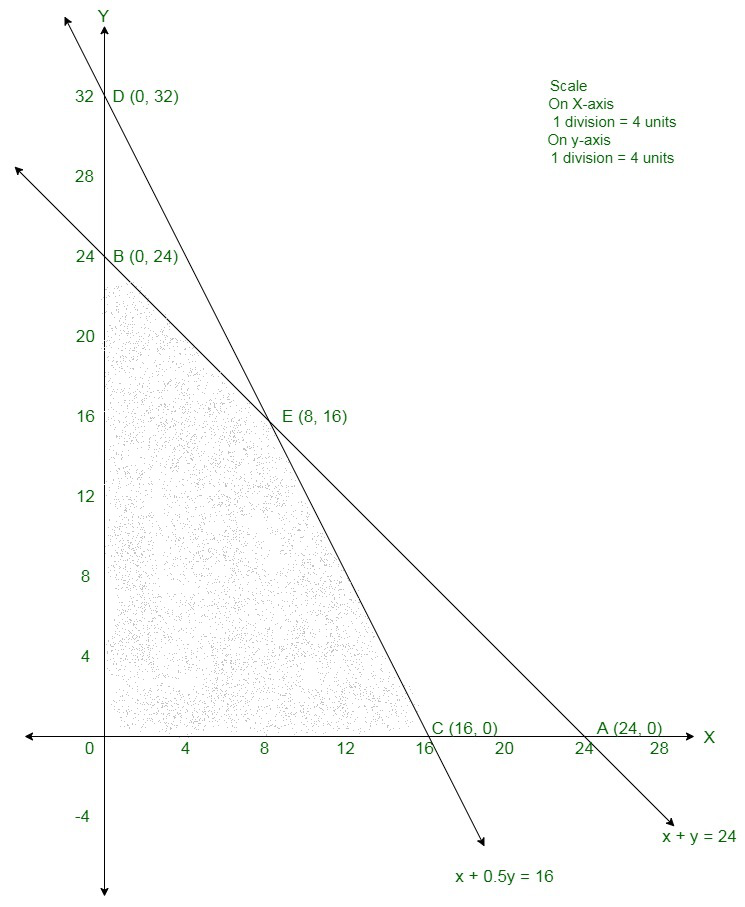The corner points are O(0, 0), C(16, 0), E(8, 16) and B(0, 24).

The value of Z at these corner points are so follows

Therefore, the maximum value of Z is 5440 at E(8, 16).

Therefore, the 8 gold rings and 16 chains should be manufactured per day to maximise the profits.

### Question 39. A library has to accommodate two different types of books on a shelf. The books are 6 cm and 4 cm thick and weigh 1 kg and 12 kg each respectively. The shelf is 96 cm long and almost can support a weight of 21 kg. How should the shelf be filled with the books of two types in order to include the greatest number of books? Make it so an LPP and solve it graphically.

Solution:

Assume x books of first type and y books of second type were accommodated. Number of books cannot be negative.

Therefore, x, y ≥ 0

As per the question, the given information can be shown as:

Therefore, the constraints are

6x + 4y ≤ 96

x + 1. 5y ≤ 21

Number of books = Z = x + y which is to be maximised

Therefore,

Therefore, the formula of the given linear programming problem is

Maximize Z = x + y

Subject to constraints

6x + 4y ≤ 96

x + 1. 5y ≤ 21

First we will convert in-equations into equations so follows:

6x + 4y = 96,

x + 1.5y = 21,

x = 0 and

y = 0

Area shows by 6x + 4y ≤ 96:

The line 6x + 4y = 96 connects the coordinate axes at A16, 0 and B(0, 24) respectively.

By connecting these points we will get the line 6x + 4y = 96.

So, (0, 0) assure the 6x + 4y = 96.

So, the area which haves the origin shows the solution set of the in-equation 6x + 4y ≤ 96.

Area shows by x + 1.5y ≤ 21:

The line x + 1.5y = 21 connects the coordinate axes at C(21, 0) and D(0, 14) respectively.

By connecting these points we will get the line x + 1.5y = 21.

So, (0, 0) assure the in-equation x + 1.5y ≤ 21.

So,the area which haves the origin shows the solution set of the in-equation x + 1.5y ≤ 21.

Area shown by x ≥ 0 and y ≥ 0:

Since, every point in the first quadrant assure these in-equations.

So, the first quadrant is the area shows by the in-equations x ≥ 0, and y ≥ 0.

The suitable area determined by the system of constraints

6x + 4y ≤ 96,

x + 1.5y ≤ 21,

x ≥ 0 and

y ≥ 0 are so follows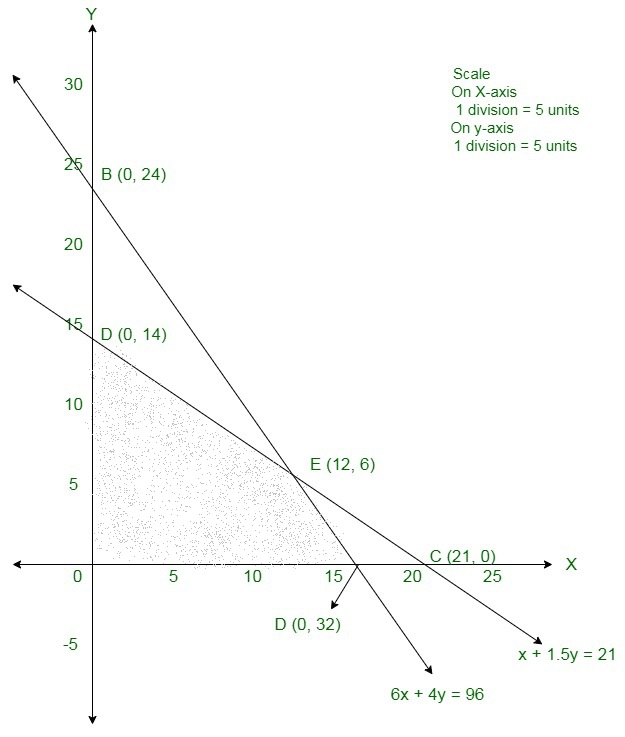The corner points are O(0, 0), D(0, 14), E(12, 6), A(16, 0) The values of Z at these corner points are so follows

The maximum value of Z is 18 which is obtained at E(12, 6).

Therefore, the maximum number of books that can be arranged on shelf is 18 where 12 books are of first type and 6 books are the other type.

### Question 40. A factory makes tennis rackets and cricket bats. A tennis racket takes 1.5 hours of machine time and 3 hours of craftman’s time in its making while a cricket bat takes 3 hours of machine time and 1 hour of craftman’s time. In a day, the factory has the availability of not more than 42 hours of machine time and 24 hours of craftman’s time. If the profit on a racket and on a bat is Rs 20 and Rs 10 respectively, find the number of tennis rackets and cricket bats that the factory must manufacture to earn the maximum profit. Make it so an LPP and solve it graphically.

Solution:

Assume x number of tennis rackets and y number of cricket bats were sold.

Number of tennis rackets and cricket balls cannot be negative.

Therefore,

x, y ≥ 0 (always)

It is given that a tennis racket takes 1.5 hours of machine time and 3 hours of craftman’s

time in its making while a cricket bat takes 3 hours of machine time and 1 hour of craftman’s time.

Also, the factory has the availability of not more than 42 hours of machine time and 24 hours of craftman’s time.

Therefore, 1. 5x + 3y ≤ 423x + y ≤ 24

If the profit on a racket and on a bat is Rs 20 and Rs 10 respectively.

Therefore, profit made on x tennis rackets and y cricket bats is Rs 20x and Rs 10y respectively.

Total profit = Z = 20x + 10y

Therefore, the formula of the given linear programming problem is

Maximize Z = 20x + 10y

Subject to constraints:

1. 5x + 3y ≤ 423

x + y ≤ 24

x ≥ 0, y ≥ 0

First we will convert in-equations into equations, so follows

1.5x + 3y = 42, 3x + y = 24, x = 0 and y = 0

Area shows by 1.5x + 3y ≤ 42:

The line 1.5x + 3y = 42 connects the coordinate axes at A(28, 0) and B(0, 14) respectively.

By connecting these points we will get the line 1.5x + 3y = 42. so, (0, 0) assure the 1.5x + 3y = 42.

So, the area which has the origin shows the solution set of the in-equation 1.5x + 3y ≤ 42.

Area shows by 3x + y ≤ 24:

The line 3x + y = 24 connects the coordinate axes at C18, 0 and D1(0, 24) respectively.

By connecting these points we will get the line 3x + y =24.

So, (0, 0) assure the in-equation 3x + y ≤ 24.

So, the area which have the origin shows the solution set of the in-equation 3x + y ≤ 24.

Area shown by x ≥ 0 and y ≥ 0:

Since, every point in the first quadrant assure these in-equations.

So, the first quadrant is the area shows by the in-equations x ≥ 0, and y ≥ 0.

The suitable area determined by the system of constraints

1.5x + 3y ≤ 42,

3x + y ≤ 24,

x ≥ 0 and

y ≥ 0 are so follows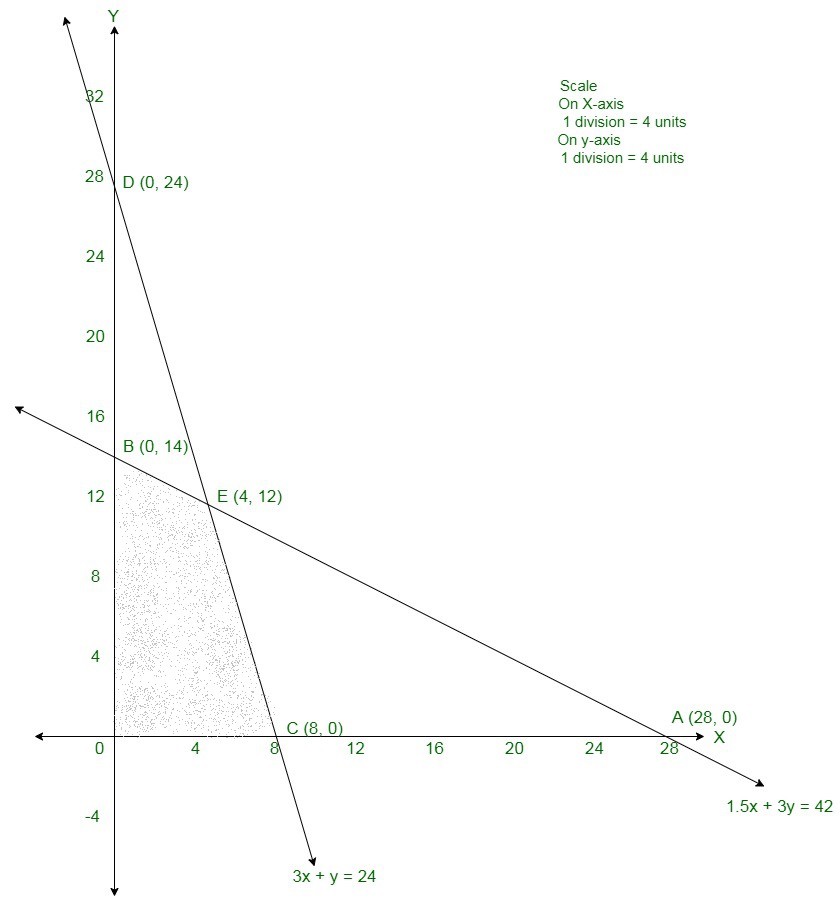In the above graph, the shaded area is the suitable area.

The corner points are O(0, 0), B(0, 14), E(4, 12), and C(8, 0).

The values of the objective function Z at corner points of the suitable area are given in the following table:

So, Z is maximum at x = 4 and y = 12 and the maximum value of Z at this point is 200.

Therefore, the maximum profit is of Rs 200 will get when 4 tennis rackets and 12 cricket bats were sold.

### Question 41. A merchant plans to sell two types of personal computers a desktop model and a portable model that will cost Rs 25,000 and Rs 40,000 respectively. He estimates that the total monthly demand of computers will not exceed 250 units. Determine the number of units of each type of computer which the merchant should stock to get maximum profit if he does not want to invest more than Rs 70 lakhs and his profit on the desktop model is Rs 4500 and on the portable model is Rs 5000. Make an LPP and solve it graphically.

Solution:

Assume x and y be the number of desktop model and portable model respectively.

Number of desktop model and portable model cannot be negative.

Therefore,

It is given that the monthly demand will not exist 250 units.

Therefore,

x + y ≤ 250

Cost of desktop and portable model is Rs 25,000 and Rs 40,000 respectively.

Therefore, cost of x desktop model and y portable model is Rs 25,000 and Rs 40,000 respectively, and he does not want to invest more than Rs 70 lakhs.

25000x + 40000y ≤ 7000000

Profit on the desktop model is Rs 4500 and on the portable model is Rs 5000.

Therefore, profit made by x desktop model and y portable model is Rs 4500x and Rs 5000y respectively.

Total profit = Z = 4500x + 5000y

Therefore, the formula of the given linear programming problem is

Maximize Z = 4500x + 5000y

Subject to constraints:

x + y ≤ 250

25000x + 40000y ≤ 7000000

x, y ≥ 0

First we will transform in-equations into equations as follows

x + y = 250,

25000x + 40000y = 7000000,

x = 0 and

y = 0

Area shown by x + y ≤ 250:

The line x + y = 250 connects the coordinate axes at A250, 0 and B(0, 250) respectively.

By connecting these points we will get the line x + y = 250.

So, (0, 0) assures the x + y = 250.

So, the Area which contains the origin shows the solution set of the in-equation x + y ≤ 250.

Area shown by 25000x + 40000y ≤ 7000000:

The line 25000x + 40000y = 7000000 connects the coordinate axes at C(280, 0) and D(0, 175) respectively.

By connecting these points we will get the line 25000x + 40000y = 7000000.

So, (0, 0) assures the in-equation 25000x + 40000y ≤ 7000000.

So, the Area which contains the origin shows the solution set of the in-equation 25000x + 40000y ≤ 7000000.

Area shown by x ≥ 0 and y ≥ 0:

Since, every point in the first quadrant assures these in-equations.

So, the first quadrant is the Area shown by the in-equations x ≥ 0, and y ≥ 0.

The suitable Area determined by the system of constraints x + y ≤ 250, 25000x + 40000y ≤ 7000000, x ≥ 0 and y ≥ 0 are as follows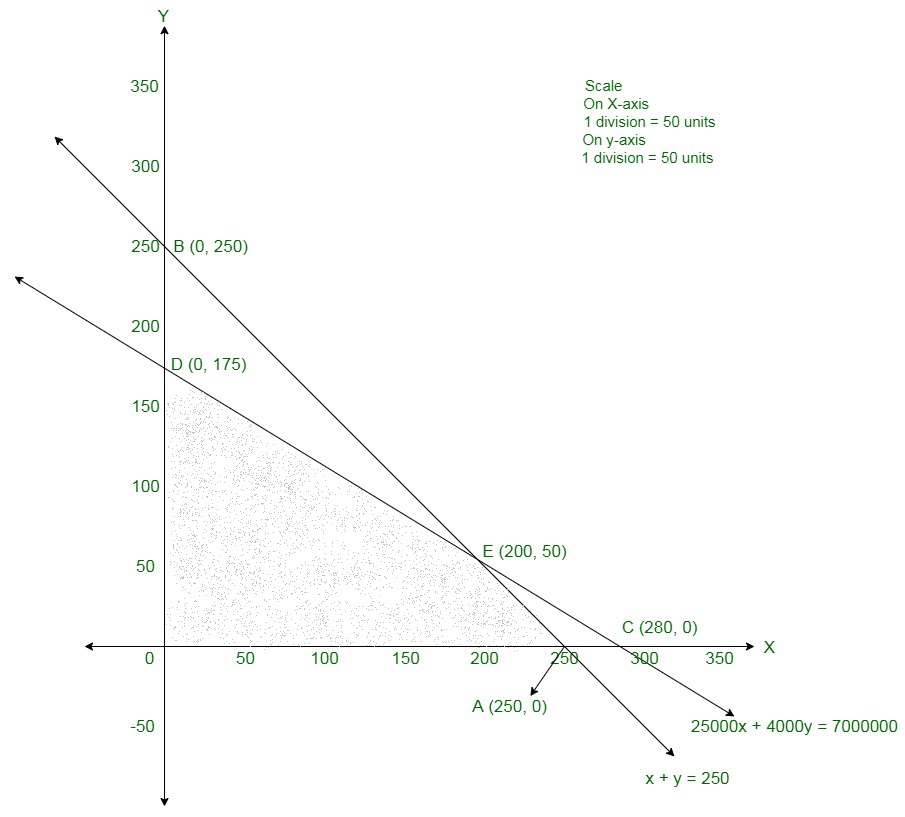The corner points are O(0, 0), D(0, 175), E(200, 50) and A(250, 0).

The values of the objective function Z at corner points of the suitable Area are given in the following table

So, Z is maximum at x = 200 and y = 50 and the maximum value of Z at this point is 1150000.

Therefore, 200 desktop models and 50 portable units should be sold to maximize the profit.

### Question 42. A cooperative society of farmers has 50 hectares of land to grow two crops X and Y. The profits from crops X and Y per hectare are estimated as 10,500 and 9,000 respectively. To control weeds, a liquid herbicide has to be used for crops X and Y at the rate of 20 litres and 10 litres per hectare, respectively. Further, not more than 800 litres of herbicide should be used in order to protect fish and wildlife using a pond that collects drainage from this land. How much land should be allocated to each crop so as to maximise the total profit of the society?

Solution:

Assume the land allocated for crop X be x hectares and crop Y be y hectares.

Maximum area of the land available for two crops is 50 hectares.

Therefore,

x + y ≤ 50

The liquid herbicide to be used for crops X and Y are at the rate of 20 litres and 10 litres per hectare, respectively.

Maximum amount of herbicide to be used is 800 litres.

Therefore,

20x + 10 y ≤ 800

⇒ 2x + y ≤ 80

The profits from crops X and Y per hectare are 10,500 and 9,000, respectively.

Therefore, total profit = (10,500x + 9,000y)

Therefore, the formula of the given linear programming problem is

Maximise Z = 10500x + 9000y

Subject to the constraints

x + y ≤ 50

2x + y ≤ 80

x ≥ 0,

y ≥ 0

The suitable Area determined by the given constraints can be diagrammatically shown as,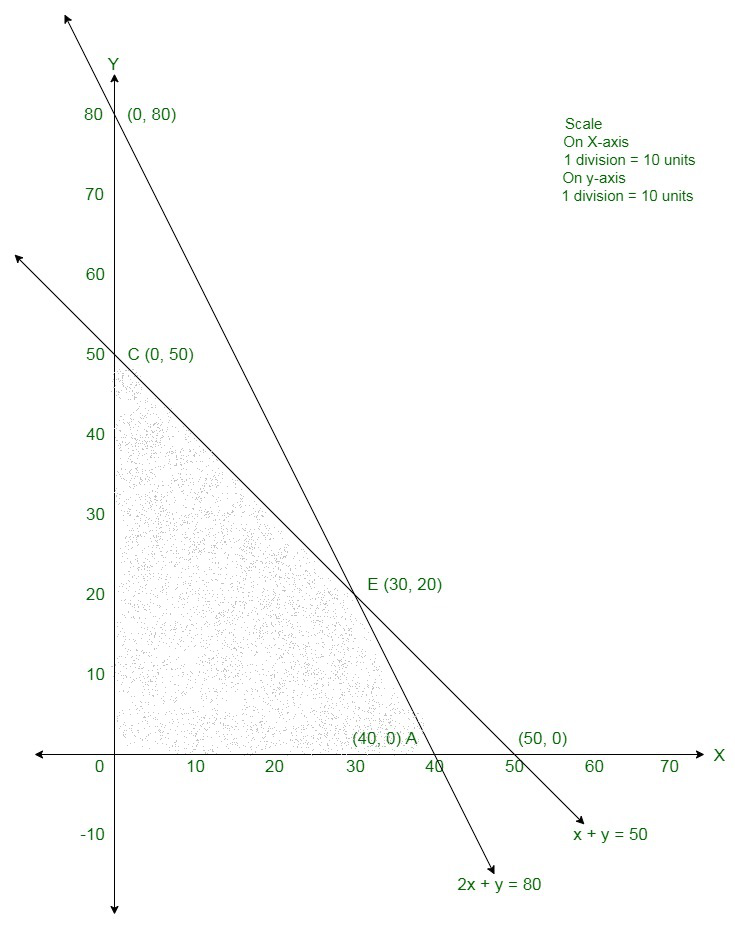The coordinates of the corner points of the suitable Area are O(0, 0), A(40, 0), B(30, 20) and C(0, 50).

The value of the objective function at these points are given in the following table

So, the maximum value of Z is 495000 at x = 30 and y = 20.

Therefore, 30 hectares of land should be allocated for crop X and 20 hectares

of land should be allocated for crop Y.

So, the maximum profit of the society is 4,95,000.

### Question 43. A manufacturing company makes two models A and B of a product. Each piece of model A requires 9 labour hours for fabricating and 1 labour hour for finishing. Each piece of model B requires 12 labour hours for fabricating and 3 labour hours for finishing. For fabricating and finishing, the maximum labour hours available are 180 and 30 respectively. The company makes a profit of 8000 on each piece of model A and 12000 on each piece of model B. How many pieces of model A and model B should be manufactured per week to realise a maximum profit? What is the maximum profit per week?

Solution:

Let x pieces of model A and y pieces of model B are manufactured per week.

Since each piece of model A requires 9 labour hours and each piece of model B requires 12 labour hours for fabricating.

Therefore, x pieces of model A and y pieces of model B require (9x + 12y) labour hours for fabricating.

But, the maximum labour hours available for fabricating are 180.

Therefore,

9x + 12y ≤ 180

⇒ 3x + 4y ≤ 60

Similarly, each piece of model A requires 1 labour hour and each piece of model B

requires 3 labour hours for finishing,

Therefore, x pieces of model A and y pieces of model B require (x + 3y) labour hours for finishing.

But, the maximum labour hours available for finishing are 30.

Therefore,

x + 3y ≤ 30

The profit from each piece of model A is 8,000 and from each piece of model B is 12,000.

Therefore, the total profit from x pieces of model A and y pieces of model B is (8,000x + 12,000y).

Therefore, the formula of the given linear programming problem is

Maximise Z = 8000x + 12000y

Subject to the constraints

3x + 4y ≤ 60

x + 3y ≤ 30

x, y ≥ 0

The suitable Area determined by the given constraints can be diagrammatically shown as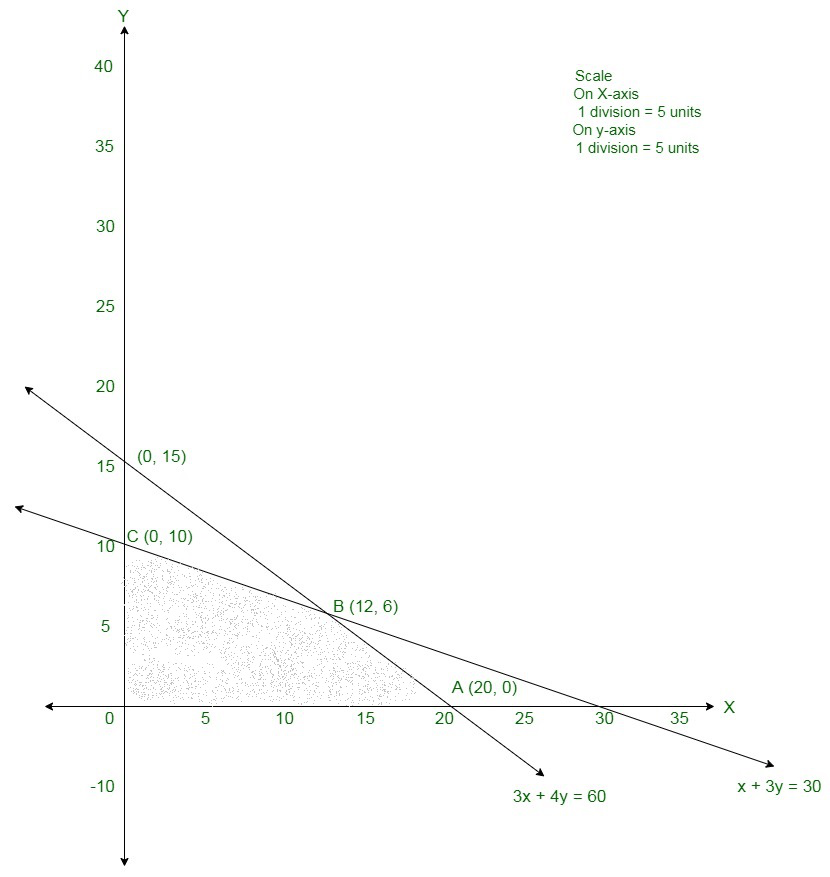The coordinates of the corner points of the suitable Area are O(0, 0), A(20, 0), B(12, 6) and C(0, 10).

The value of the objective function at these points are given in the following table.

The maximum value of Z is 168000 at x = 12, y = 6.

Therefore, the manufacturing company should produce 12 pieces of model A and

6 pieces of model B to realise maximum profit.

So, the maximum profit is 1,68,000.

### Question 44. A factory makes tennis rackets and cricket bats. A tennis racket takes 1.5 hours of machine time and 3 hours of craftman’s time in its making while a cricket bat takes 3 hours of machine time and 1 hour of craftman’s time. In a day, the factory has the availability of not more than 42 hours of machine time and 24 hours of craftman’s time.

(i) What number of rackets and bats must be made if the factory is to work at full capacity?

(ii) If the profit on a racket and on a bat is Rs 20 and Rs 10 respectively, find the maximum profit of the factory when it works at full capacity.

Solution:

Assume x number of tennis rackets and y number of cricket bats were sold.

Number of tennis rackets and cricket balls cannot be negative.

Therefore,

x ≥ 0, y ≥ 0

It is given that a tennis racket takes 1.5 hours of machine time and 3 hours of craftman’s time

in its making while a cricket bat takes 3 hours of machine time and 1 hour of craftman’s time.

Also, the factory has the availability of not more than 42 hours of machine time and 24 hours of craftman’s time.

Therefore, 1 · 5x + 3y ≤ 423x + y ≤ 24

If the profit on a racket and on a bat is Rs 20 and Rs 10 respectively.

Therefore, profit made on x tennis rackets and y cricket bats is Rs 20x and Rs 10y respectively.

Total profit = Z = 20x + 10y

Therefore, the formula of the given linear programming problem is

Maximize Z = 20x + 10y

Subject to constraints:

1 · 5x + 3y ≤ 42

3x + y ≤ 24

x ≥ 0, y ≥ 0

First we will transform in-equations into equations as follows:

1.5x + 3y = 42,

3x + y = 24,

x = 0 and

y = 0

Area shown by 1.5x + 3y ≤ 42:

The line 1.5x + 3y = 42 connects the coordinate axes at A(28, 0) and B(0, 14) respectively.

By connecting these points we will get the line 1.5x + 3y = 42.

So, (0, 0) assures the 1.5x + 3y = 42.

So, the Area which contains the origin shows the solution set of the in-equation 1.5x + 3y ≤ 42.

Area shown by 3x + y ≤ 24:

The line 3x + y = 24 connects the coordinate axes at C(8, 0) and D(0, 24) respectively.

By connecting these points we will get the line 3x + y = 24. So, 0, 0 assures the in-equation 3x + y ≤ 24.

So, the Area which contains the origin shows the solution set of the in-equation 3x + y ≤ 24.

Area shown by x ≥ 0 and y ≥ 0:

Since, every point in the first quadrant assures these in-equations.

So, the first quadrant is the Area shown by the in-equations x ≥ 0, and y ≥ 0.

The suitable Area determined by the system of constraints

1.5x + 3y ≤ 42,

3x + y ≤ 24,

x ≥ 0 and

y ≥ 0 are as follows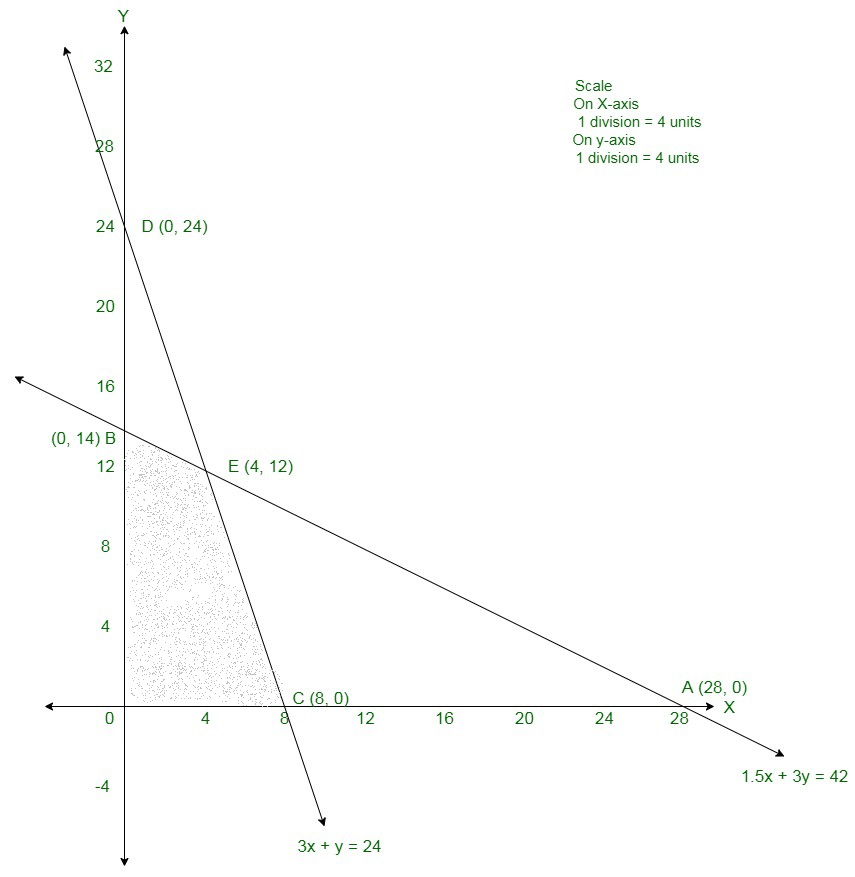In the above graph, the shaded Area is the suitable Area.

The corner points are O(0, 0), B(0, 14), E(4, 12), and C(8, 0).

The values of the objective function Z at corner points of the suitable Area are given in the following table

So, Z is maximum at x = 4 and y = 12 and the maximum value of Z at this point is 200.

(i) 4 tennis rackets and 12 cricket bats must be made if the factory is to work at full capacity.

(ii) The maximum profit of the factory when it works at full capacity is Rs 200.

### Question 45. A merchant plans to sell two types of personal computers a desktop model and a portable model that will cost Rs 25,000 and Rs 40,000 respectively. He estimates that the total monthly demand of computers will not exceed 250 units. Determine the number of units of each type of computers which the merchant should stock to get maximum profit if he does not want to invest more than Rs 70 lakhs and his profit on the desktop model is Rs 4500 and on the portable model is Rs 5000.

Solution:

Assume x and y be the number of desktop model and portable model respectively.

Number of desktop model and portable model cannot be negative.

Therefore,

x, y ≥ 0 (always)

It is given that the monthly demand will not exist 250 units.

Therefore, x + y ≤ 250

Cost of desktop and portable model is Rs 25,000 and Rs 40,000 respectively.

Therefore, cost of x desktop model and y portable model is Rs 25,000 and Rs 40,000 respectively and

he does not want to invest more than Rs 70 lakhs.

25000x + 40000y ≤ 7000000

Profit on the desktop model is Rs 4500 and on the portable model is Rs 5000.

Therefore, profit made by x desktop model and y portable model is Rs 4500x and Rs 5000y respectively.

Total profit = Z = 4500x + 5000y

Therefore, the formula of the given linear programming problem is

Maximize Z = 4500x + 5000y

Subject to constraints:

x + y ≤ 250

25000x + 40000y ≤ 7000000

x, y ≥ 0

First we will transform in-equations into equations as follows:

x + y = 250,

25000x + 40000y = 7000000,

x = 0 and

y = 0

Area shown by x + y ≤ 250:

The line x + y = 250 connects the coordinate axes at A(250, 0) and B(0, 250) respectively.

By connecting these points we will get the linex + y = 250.

So, 0, 0 assures the x + y = 250.

So, the Area which contains the origin shows the solution set of the in-equation x + y ≤ 250.

Area shown by 25000x + 40000y ≤ 7000000:

The line 25000x + 40000y = 7000000 connects the coordinate axes at C(280, 0) and D(0, 175) respectively.

By connecting these points we will get the line 25000x + 40000y = 7000000.

So, (0, 0) assures the in-equation 25000x + 40000y ≤ 7000000.

So, the Area which contains the origin shows the solution set of the in-equation 25000x + 40000y ≤ 7000000.

Area shown by x ≥ 0 and y ≥ 0:

Since, every point in the first quadrant assures these in-equations.

So, the first quadrant is the Area shown by the in-equations x ≥ 0, and y ≥ 0.

The suitable Area determined by the system of constraints x + y ≤ 250, 25000x + 40000y ≤ 7000000, x ≥ 0 and y ≥ 0 are as followsThe corner points are O(0, 0), D(0, 175), E(200, 50) and A(250, 0).

The values of the objective function Z at corner points of the suitable Area are given in the following table:

So, Z is maximum at x = 200 and y = 50 and the maximum value of Z at this point is 1150000.

Therefore, 200 desktop models and 50 portable units should be sold to maximize the profit.

### Question 46. A toy company manufactures two types of dolls, A and B. Market tests and available resources have indicated that the combined production level should not exceed 1200 dolls per week and the demand for dolls of type B is at most half of that for dolls of type A. Further, the production level of dolls of type A can exceed three times the production of dolls of other type by at most 600 units. If the company makes profit of 12 and 16 per doll respectively on dolls A and B, how many of each should be produced weekly in order to maximise the profit?

Solution:

Assume x units of doll A and y units of doll B are manufactured to will get the maximum profit.

The mathematical formulation of the above problem is as follows:

Maximize Z = 12x + 16y Subject to

x + y ≤ 1200y ≤ x/12,

x – 3y ≤ 600,

x, y ≥ 0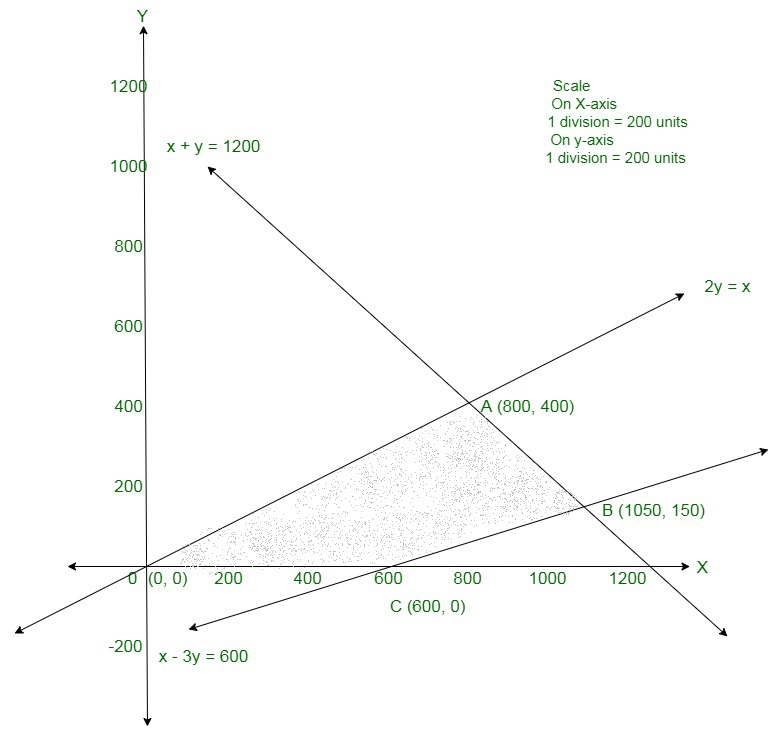The shaded Area shows the set of suitable solutions.

The coordinates of the corner points of the suitable Area are O(0, 0), A(800, 400), B(1050, 150) and C(600, 0).

The value of Z at O0, 0 = 12(0) + 16(0) = 0

The value of Z at A800, 400 = 12(800) + 16(400) = 16000 Maximum

The value of Z at B1050, 150 = 12(1050) + 16(150) = 15000

The value of Z at C600, 0 = 12(600) + 16(0) = 7200

Therefore, 800 units of doll A and 400 units of doll B should be

produced weekly to get the maximum profit of Rs 16000.

### Question 47. There are two types of fertilizers F1 and F2. F1 consists of 10% nitrogen and 6% phosphoric acid and F2 consists of 5% nitrogen and 10% phosphoric acid. After testing the soil conditions, a farmer finds that she needs at least 14 kg of nitrogen and 14 kg of phosphoric acid for her crop. If F1 costs 6/kg and F2 costs 5/kg, determine how much of each type of fertilizer should be used so that the nutrient requirements are met at minimum cost. What is the minimum cost?

Solution:

Let x kg of fertilizer F1 and y kg of fertilizer F2 is used to meet the nutrient requirements.

F1 consists of 10% nitrogen and F2 consists of 5% nitrogen.

But, the farmer needs atleast 14 kg of nitrogen for the crops.

Therefore,

10% of x kg + 5% of y kg ≥ 14 kg

=> x/10 + y/20 ≥ 14

=> 2x + y ≥ 280

Similarly, F1 consists of 6% phosphoric acid and F2 consists of 10% phosphoric acid.

But, the farmer needs atleast 14 kg of phosphoric acid for the crops.

Therefore,

6% of x kg + 10% of y kg ≥ 14 kg

=> 6x/100 + 10y/200 ≤ 14

=> 3x + 5y ≤ 700

The cost of fertilizer F1 is 6/kg and fertilizer F2 is 5/kg,

Therefore, total cost of x kg of fertilizer F1 and y kg of fertilizer F2 is (6x + 5y).

Therefore, the formula of the given linear programming problem is

Minimise Z = 6x + 5y

Subject to the constraints:

2x + y ≥ 280

3x + 5y ≥ 700

x, y ≥ 0

The suitable Area determined by the given constraints can be diagrammatically shown as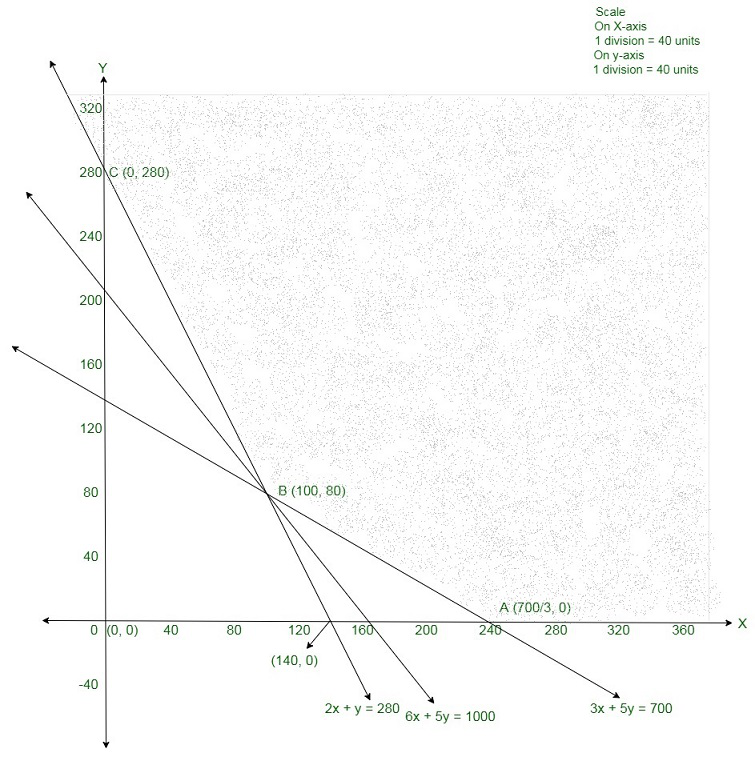The coordinates of the corner points of the suitable Area are A(700/3, 0) , B(100, 80) and C(0, 280).

The value of the objective function at these points are given in the following table.

The smallest value of Z is 1000 which is acquired at x = 100, y = 80.

It can be seen that the open half-plane shown by 6x + 5y < 1000 has no common points with the suitable Area.

So, the minimum value of Z is 1000.

Therefore, 100 kg of fertilizer F1 and and 80 kg of fertilizer F2 should be used so that the nutrient requirements are met at minimum cost.

So, the minimum cost is 1,000.

### She makes a profit of 600 and 400 on items M and N respectively. How many of each item should she produce so as to maximise her profit assuming that she can sell all the items that she produced? What will be the maximum profit?

Solution:

Let x units of item M and y units of item N are produced to maximise the profit.

Since each unit of item M require 1 hours on machine I and each unit of item N require 2 hours on machine I, therefore, the total hours required for producing

x units of item M and y units of item N on machine I are (2x + y).

But, machines I is capable of being operated for at most 12 hours.

Therefore,

2x + y ≤ 12

Similarly, each unit of item M require 2 hours on machine II and each unit

of item N require 1 hour on machine II, therefore, the total hours required for

producing x units of item M and y units of item N on machine II are (x + 2y).

But, machines II is capable of being operated for at most 12 hours.

Therefore,

x + 2y ≤ 12

Also, each unit of item M require 1 hour on machine III and each unit of item N

require 1.25 hour on machine III, therefore, the total hours required for producing

x units of item M and y units of item N on machine III are (x + 1.25y).

But, machines III must be operated for atleast 5 hours.

Therefore,

x + 1.25y ≥ 5

The profit from each unit of item M is 600 and each unit of item N is 400.

Therefore, the total profit from x units of item M and y units of item N is (600x + 400y).

Therefore, the formula of the given linear programming problem is

Maximise Z = 600x + 400y

Subject to the constraints:

2x + y ≤ 12

x + 2y ≤ 12

x + 1.25y ≥ 5

x, y ≥ 0

The suitable Area determined by the given constraints can be diagrammatically shown as,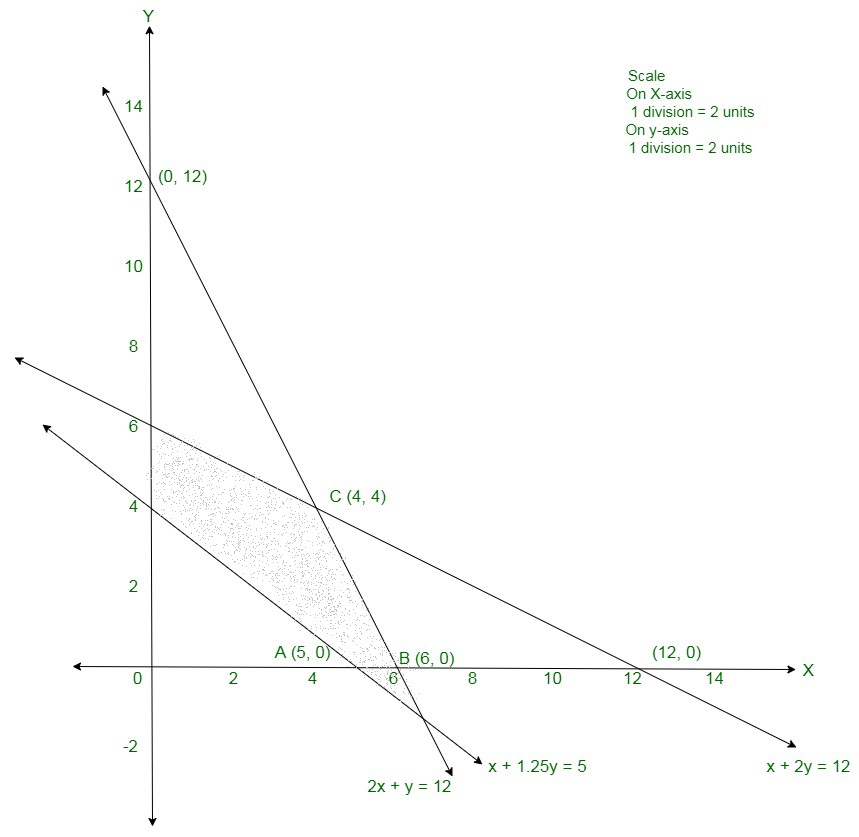The coordinates of the corner points of the suitable Area are A(5, 0), B(6, 0), C(4, 4), D(0, 6) and E(0, 4).

The value of the objective function at these points are given in the following table.

The maximum value of Z is 4000 at x = 4, y = 4.

Therefore, 4 units of item M and 4 units of item N should be produced to maximise the profit.

So, the maximum profit of the manufacturer is 4,000.

### How many units should be transported from each factory to each depot in order that the transportation cost is minimum. What will be the minimum transportation cost?

Solution:

Here, demand of the commodity 5 + 5 + 4 = 14units is equal the supply of the commodity 8 + 6 = 14units.

So, no commodity would be left at the two factories.

Assume x units and y units of the commodity be transported from the factory P to the depots at A and B, respectively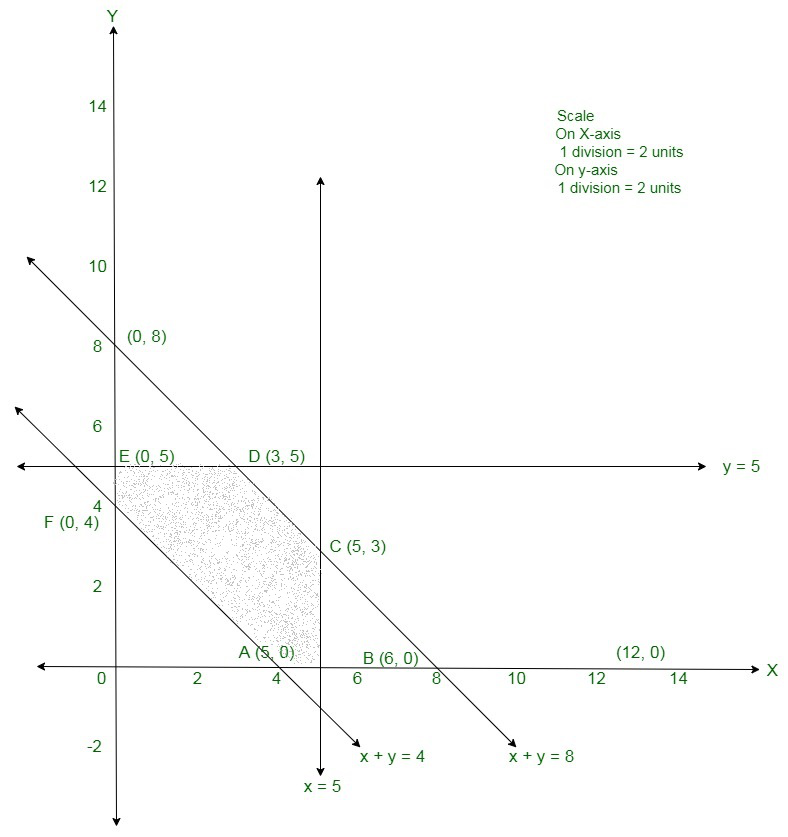Then, (8 − x − y) units of the commodity will be transported from the factory P to the depot C.

Now, the weekly requirement of depot A is 5 units of the commodity.

Now, x units of the commodity are transported from factory P, so

the remaining (5 − x) units of the commodity are transported from

the factory Q to the depot A.

The weekly requirement of depot B is 5 units of the commodity.

Now, y units of the commodity are transported from factory P,

so the remaining (5 − y) units of the commodity are transported

from the factory Q to the depot B.

Similarly, 6 − (5 − x) − (5 − y) = (x + y − 4) units of the commodity will

be transported from the factory Q to the depot C.

Since the number of units of commodity transported are from the

factories to the depots are non-negative. Therefore,

x ≥ 0, y ≥ 0, 8 − x − y ≥ 0, 5 − x ≥ 0, 5 − y ≥ 0, x + y − 4 ≥ 0

Or

x ≥ 0, y ≥ 0, x + y ≤ 8, x ≤ 5, y ≤ 5, x + y ≥ 4

Total transportation cost = 160x + 100y + 150(8 − x − y) + 100(5 − x) +

120(5 − y) + 100(x + y − 4) = 10x − 70y + 1900

Therefore, the formula of the given linear programming problem is

Minimise Z = 10x − 70y + 1900

Subject to the constraints:

x + y ≤ 8

x ≤ 5

y ≤ 5

x + y ≥ 4

x ≥ 0, y ≥ 0

The suitable Area determined by the given constraints can be diagrammatically shown as,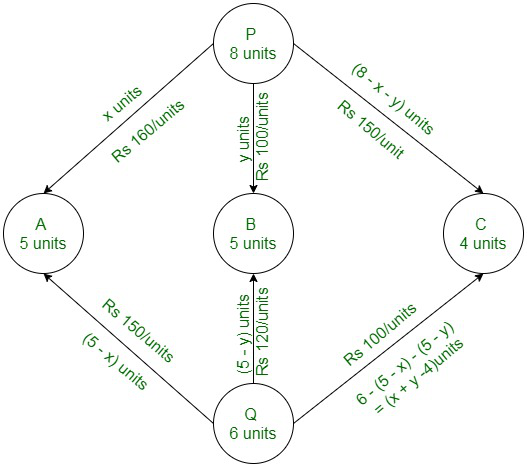The coordinates of the corner points of the suitable Area are A(4, 0), B(5, 0), C(5, 3), D(3, 5), E(0, 5) and F(0, 4).

The value of the objective function at these points are given in the following table.

The minimum value of Z is 1550 at x = 0, y = 5.

Therefore, the minimum transportation cost, factory P should supply 0, 5, 3 units of commodity

to depots A, B, C respectively and factory Q should supply 5, 0, 1 units of commodity to depots

A, B, C respectively.

So, the minimum transportation cost is 1,550.

### Each machine is available for a maximum of 6 hours per day. If the profit on each toy of type A is 7.50 and that on each toy of type B is 5, show that 15 toys of type A and 30 toys of type B should be manufactured in a day to get maximum profit.

Solution:

Let the manufacturer makes x toys of type A and y toys of type B.

Since each toy of type A require 12 minutes on machine I and

each toy of type B require 6 minutes on machine I, therefore, x toys of typ

A and y toys of type B require (12x + 6y) minutes on machine I.

But, machines I is available for at most 6 hours.

Therefore,

12x + 6y ≤ 360

⇒ 2x + y ≤ 60

Similarly, each toy of type A require 18 minutes on machine II and

each toy of type B require 0 minutes on machine II, therefore,

x toys of type A and y toys of type B require (18x + 0y) minutes on machine II.

But, machines II is available for at most 6 hours.

Therefore,

18x + 0y ≤ 360

⇒ x ≤ 20

Also, each toy of type A require 6 minutes on machine III and

each toy of type B require 9 minutes on machine III,

therefore, x toys of type A and y toys of type B require (6x + 9y) minutes

on machine III. But, machines III is available for at most 6 hours.

Therefore,

6x + 9y ≤ 360

⇒ 2x + 3y ≤ 120

The profit on each toy of type A is 7.50 and each toy of type B is 5. Therefore, the total profit from x toys of type A and y toys of type B is (7.50x + 5y).

Therefore, the formula of the given linear programming problem is

Maximise Z = 7.5x + 5y

Subject to the constraints:

2x + y ≤ 60

x ≤ 20

2x + 3y ≤ 120

x, y ≥ 0

The suitable Area determined by the given constraints can be diagrammatically shown as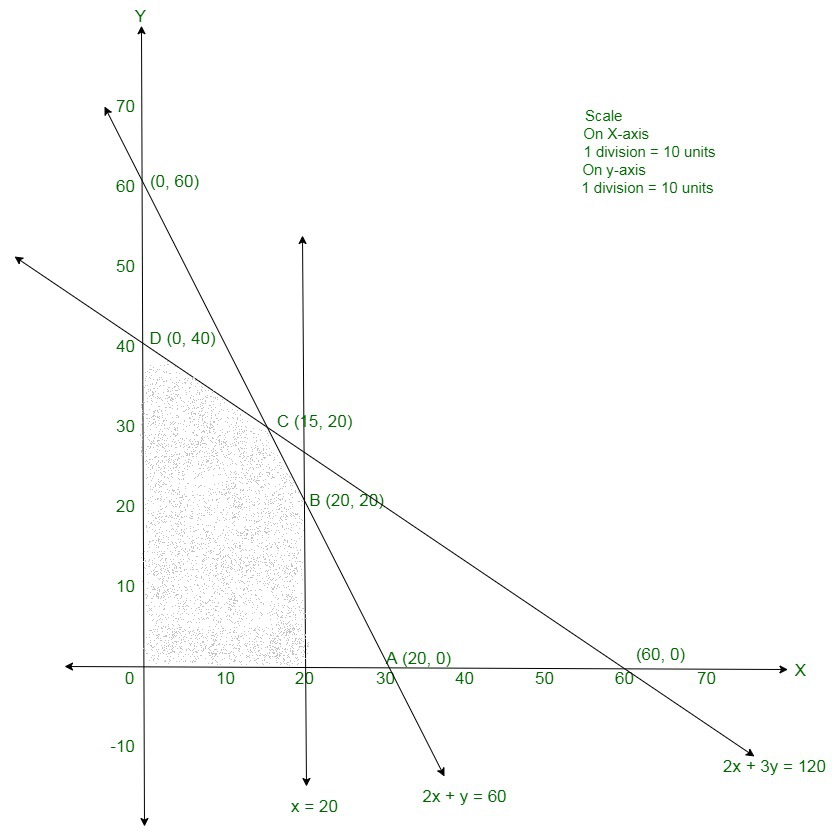The coordinates of the corner points of the suitable Area are O(0, 0), A(20, 0), B(20, 20), C(15, 30) and D(0, 40).

The value of the objective function at these points are given in the following table.

The maximum value of Z is 262.5 at x = 15, y = 30.

Therefore, 15 toys of type A and 30 toys of type B should be manufactured in a day to get maximum profit.

So, the maximum profit is 262.50

### Question 51. An aeroplane can carry a maximum of 200 passengers. A profit of 1000 is made on each executive class ticket and a profit of 600 is made on each economy class ticket. The airline reserves atleast 20 seats for the executive class. However, atleast 4 times as many passengers prefer to travel by economy class than by the executive class. Determine how many tickets of each type must be sold in order to maximize the profit of the airline. What is the maximum profit?

Solution:

Assume x tickets of executive class and y tickets of economy class are sold by the airline.

The profit on each executive class ticket is 1000 and on each economy class ticket is 600.

Thus, the total profit from x executive class tickets and y economy class ticket is (1000x + 600y).

Now here, the aeroplane can carry a maximum of 200 passengers.

Therefore,

x + y ≤ 200

The airline reserves atleast 20 seats for executive class.

Therefore,

x ≥ 20

Also, at minimum 4 times as many passengers prefer to travel by economy class than by the executive class.

Therefore,

y ≥ 4x

Therefore, the formula of the given linear programming problem is

Maximise Z = 1000x + 600y

Subject to the constraints:

x + y ≤ 200

x ≥ 20

y ≥ 4x

x, y ≥ 0

The Suitable area determined by the given constraints can be diagrammatically shown as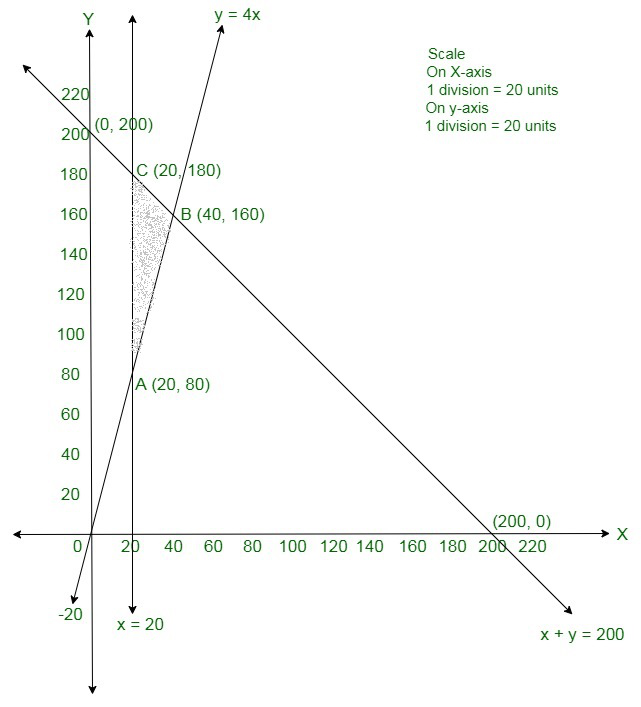The coordinates of the corner points of the feasible region are A(20, 80), B(40, 160) and C(20, 180).

The value of the objective function at these points are given in the following table

The maximum value of Z is 136000 at x = 40, y = 160.

Thus, 40 tickets of executive class and 160 tickets of economy class should be sold to maximise the profit.

So, the maximum profit of the airline is 1,36,000.

### Question 52. A manufacturer considers that men and women workers are equally efficient and so he pays them at the same rate. He has 30 and 17 units of workers male and female and capital respectively, which he uses to produce two types of goods A and B. To produce one unit of A, 2 workers and 3 units of capital are required while 3 workers and 1 unit of capital is required to produce one unit of B. If A and B are priced at 100 and 120 per unit respectively, how should he use his resources to maximize the total revenue? Form the above as an LPP and solve graphically. Do you agree with this view of the manufacturer that men and women workers are equally efficient and so should be paid at the same rate?

Solution:

Assume x units of A and y units of B be produced by the manufacturer.

The price of one unit of A is 100 and the price of one unit of B is 120.

Thus, the total price of x units of A and y units of B or the total revenue is (100x + 120y).

One unit of A needs 2 workers and one unit of B needs 3 workers.

Hence, x units of A and y units of B needs (2x + 3y) workers.

But, the manufacturer has 30 workers.

Therefore,

2x + 3y ≤ 30

Similarly, one unit of A needs 3 units of capital and one unit of B needs 1 unit of capital.

Thus, x units of A and y units of B needs (3x + y) units of capital.

But, the manufacturer has 17 units of capital.

Therefore,

3x + y ≤ 17

Hence, the given linear programming problem is

Maximise Z = 100x + 120y

Subject to the constraints:

2x + 3y ≤ 30

3x + y ≤ 17

x, y ≥ 0

The suitable area determined by the given constraints can be diagrammatically shown as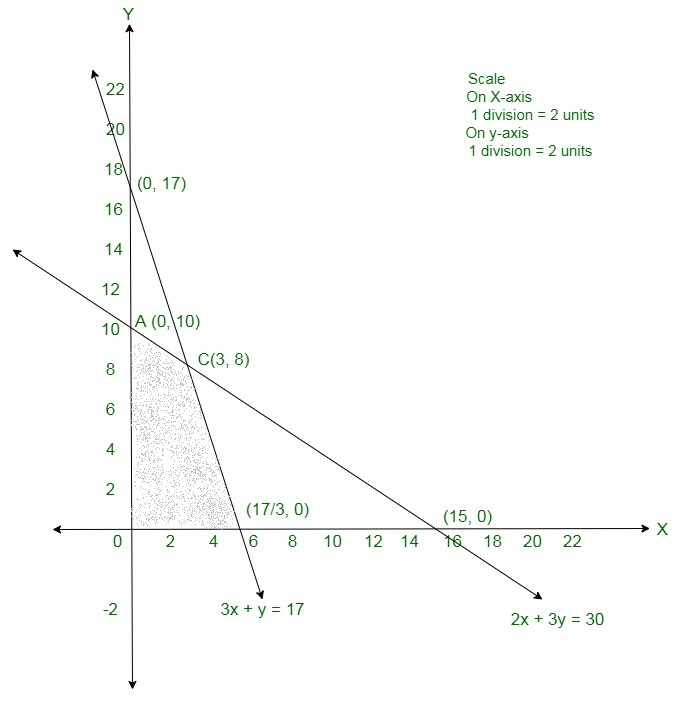The coordinates of the corner points of the feasible region are O(0, 0), A(0, 10), B (17/3, 0) and C(3, 8).

The value of the objective function at these points are showing the following table.

The maximum value of Z is 1260 at x = 3, y = 8.

Therefore, the maximum total revenue is 1,260 when 3 units of A and 8 units of B are produced.

Yes, because the efficiency of a worker does not depend on whether the worker is a male or a female.

My Personal Notes arrow_drop_up